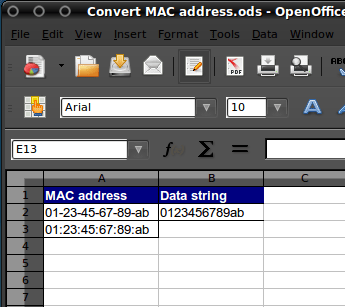## Cleaning phone numbers in Excel or OO Spreadsheet

Personally I’ve been using Excel for storing phone numbers for almost twenty years now. Every now and then I don’t recognize the phone number so I need to search in my Excel sheet looking up who called me. After ten years and 500 phone numbers plus, the information stored in the Excel sheet is ‘poluted’ with hyphens and spaces. So in this article I’ll try to explain how to clean up messy telephone numbers by removing spaces and separators in Excel?

Posted on

As I’m currently working a lot with Excel, I get a lot of questions like “How do I do this?” or “How do I do that?”. Yesterday someone asked me how to convert a data string to a MAC address. The standard format for printing MAC addresses in human-friendly form is six groups of two hexadecimal digits, separated by hyphens (-) or colons (:), in transmission order, e.g. 01-23-45-67-89-ab, 01:23:45:67:89:ab. In this article I’ll give you examples of how to add, remove or change the separator for MAC addresses in MS Excel or OO Spreadsheet.

Adding a seperator (change 0123456789ab into 01-23-45-67-89-ab)=CONCATENATE(MID(A2;1;2);”-“;MID(A2;3;2);”-“;MID(A2;5;2);”-“;MID(A2;7;2);”-“;MID(A2;9;2);”-“;MID(A2;11;2)) =CONCATENATE(MID(A2;1;2);”:”;MID(A2;3;2);”:”;MID(A2;5;2);”:”;MID(A2;7;2);”:”;MID(A2;9;2);”:”;MID(A2;11;2))

An alternate solution was send in by Franco Magliaro see below:

 =MID(A2,1,2)&”-“&MID(A2,3,2)&”-“&MID(A2,5,2)&”-“&MID(A2,7,2)&”-“&MID(A2,9,2)&”-“&MID(A2,11,2) =MID(A2,1,2)&”:”&MID(A2,3,2)&”:”&MID(A2,5,2)&”:”&MID(A2,7,2)&”:”&MID(A2,9,2)&”:”&MID(A2,11,2)

Removing a separator (change 01-23-45-67-89-ab into 0123456789ab)=CONCATENATE(MID(A2;1;2);MID(A2;4;2);MID(A2;7;2);MID(A2;10;2);MID(A2;13; 2);MID(A2;16;2))

or the easy way

 =SUBSTITUTE(A2;”-“;””) =SUBSTITUTE(A2;”:”;””)

Changing a separator (change 01-23-45-67-89-ab into 01:23:45:67:89:ab)=CONCATENATE(MID(A2;1;2);”:”;MID(A2;4;2);”:”;MID(A2;7;2);”:”;MID(A2;10;2 );”:”;MID(A2;13;2);”:”;MID(A2;16;2)) =CONCATENATE(MID(A3;1;2);”-“;MID(A3;4;2);”-“;MID(A3;7;2);”-“;MID(A3;10;2 );”-“;MID(A3;13;2);”-“;MID(A3;16;2))

or the easy way 🙂

 =SUBSTITUTE(A2;”-“;”:”) =SUBSTITUTE(A2;”:”;”-“)

An alternate solution, to convert the Cisco 0123.4567.89ab MAC format to the F5 load balancer MAC format of 01:23:45:67:89:ab, was send in by Mike Wintrode. To add an extra twist, the F5 load balancer also removes the leading “0” in any pair, so for instance 00:14:0F:80:a3:01 would show as 0:14:f:80:a3:1.

So Mike created the following:

 =CONCATENATE(IF(MID(\$A2,1,1)=”0″,MID(\$A2,2,1),MID(\$A2,1,2)),”:”,IF(MID(\$A2,3,1)=”0″,MID(\$A2,4,1),MID(\$A2,3,2)),”:”,IF(MID(\$A2,6,1)=”0″,MID(\$A2,7,1),MID(\$A2,6,2)),”:”,IF(MID(\$A2,8,1)=”0″,MID(\$A2,9,1),MID(\$A2,8,2)),”:”,IF(MID(\$A2,11,1)=”0″,MID(\$A2,12,1),MID(\$A2,11,2)),”:”,IF(MID(\$A2,13,1)=”0″,MID(\$A2,14,1),MID(\$A2,13,2)))

The basic premise utilizes an “IF” statement to check for a preceding “0” and if present to return the next character, and if not to return both characters.

Adam Heath was so kind to send me an Exel template which uses most of the above formulas